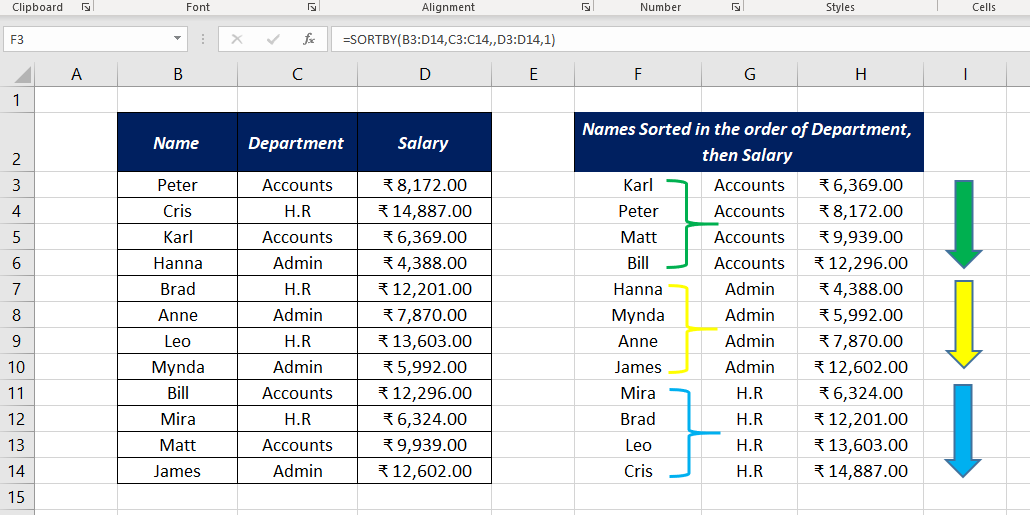# SORTBY FunctionThe Excel SORTBY function is a DYNAMIC ARRAY FUNCTION that can sort the contents of an array or range based on the values from another range or array. The array or range used to sort need not appear in the result.

### Function Type

Lookup and reference

### Purpose

Sort an array or range

Sorted array

### Syntax

`=SORTBY (array, by_array, [sort_order], [array/order], ...)`

### Arguments

array – Array or range to sort

by_array – Array or range to sort by

sort_order – [optional] Sort order, 1 for Ascending order (default) and -1 for Descending order

array/order – [optional] Additional array and sort order pairs

The main difference between SORT and SORTBY functions is that the latter supports multiple levels of sorting.

### Examples

#### Example 1

In this example, SORTBY function is used to sort a list containing the names of some students and their marks. Names are in column B (B3:B12) and corresponding marks are in Column C (C3:C12). The following formula will sort the list of students in the Ascending Order of marks.

`=SORTBY(B3:C12,C3:C12)`

Here the SORTBY function has sorted and returned the entire array as I have used the entire array (B3:C12) as the first argument. If you want the SORTBY function to return only the names, use the address of that particular array (B3:B12) in the place of the first argument.

`=SORTBY(B3:B12,C3:C12)`

To sort the list in the Descending Order of marks scored, use -1 in the place of the third argument, sort_order

`=SORTBY(B3:C12,C3:C12,-1)`

#### Example 2

Let’s see an example of performing multiple levels of sorting using the SORTBY function.

At first, we do a single level of sorting. To sort the list of employees (B3:D14) according to their salary, use the following formula.

`=SORTBY(B3:D14,D3:D14)`

Now, 2 levels of sorting using the SORTBY function.

To sort the list based on the departments and then salary use the following formula.

`=SORTBY(B3:D14,C3:C14,,D3:D14,1)`

Employee list is sorted according to their departments called Accounts, Admin and H.R. In each department employee list sorted on Ascending of salary.